Courses

# Multiplexers

## 14 Questions MCQ Test RRB JE for Computer Science Engineering | Multiplexers

Description
This mock test of Multiplexers for Computer Science Engineering (CSE) helps you for every Computer Science Engineering (CSE) entrance exam. This contains 14 Multiple Choice Questions for Computer Science Engineering (CSE) Multiplexers (mcq) to study with solutions a complete question bank. The solved questions answers in this Multiplexers quiz give you a good mix of easy questions and tough questions. Computer Science Engineering (CSE) students definitely take this Multiplexers exercise for a better result in the exam. You can find other Multiplexers extra questions, long questions & short questions for Computer Science Engineering (CSE) on EduRev as well by searching above.
QUESTION: 1

### Consider the following Boolean function of four variables: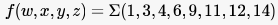The function is

Solution:

The K-map would be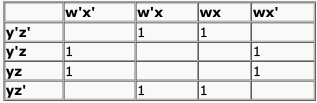So, the minimized expression would
be x'z + xz'.
So, option B.

QUESTION: 2

### Consider the following Boolean function of four variables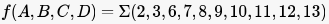The function is

Solution: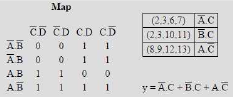QUESTION: 3

### The simplified SOP (Sum of Product) from the Boolean expression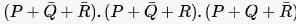1s

Solution: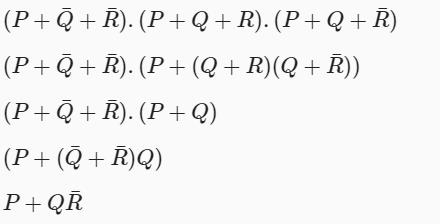QUESTION: 4

Consider the 4-to-1 multiplexer with two select lines S1 and S0 given below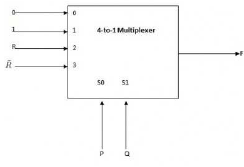The minimal sum-of-products form of the Boolean expression for the output F of the multiplexer is

Solution:

S0 and S1 are used to select the input given to be given as output.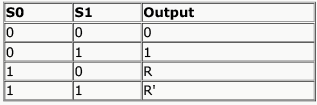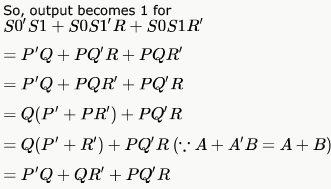QUESTION: 5

Consider the following Boolean expression for F: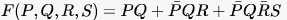The minimal sum of products form of F is

Solution: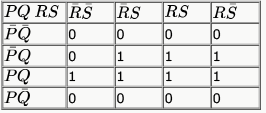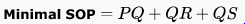Hence, option A is correct.

QUESTION: 6

Consider the following minterm expression F for :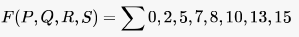The minterms 2, 7, 8 and 13 are 'do not care' terms. The minimal sum-of-products form for F is

Solution:

While putting the terms to K-map the 3rd and 4th columns are swapped so do 3rd and 4th rows. So, term 2 is going to (0,3) column instead of (0,2), 8 is going to (3,0) instead of (2,0) etc..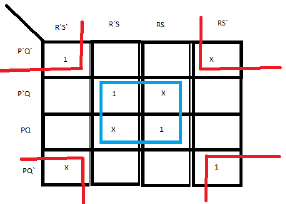Solving this k-map gives B) as the answer.

QUESTION: 7

Consider the two cascade 2 to 1 multiplexers as shown in the figure .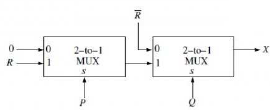The minimal sum of products form of the output X is

Solution: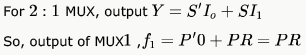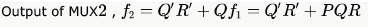QUESTION: 8

The output F of the below multiplexer circuit can be represented by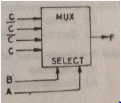Solution: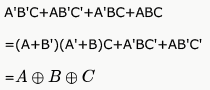QUESTION: 9

A multiplexer with a 4 - bit data select input is a

Solution:

for n bit data select inputQUESTION: 10

It is possible for an enable or strobe input to undergo an expansion of two or more MUX ICs to the digital multiplexer with the proficiency of large number of

Solution:

It is possible for an enable or strobe input to undergo an expansion of two or more MUX ICs to the digital multiplexer with the proficiency of large number of inputs.

QUESTION: 11

Consider a multiplexer with X and Y as data inputs and Z the as control input. Z = 0 selects input X, and Z = 1 selects input Y. What are the connections required to realize the 2-variable Boolean function F = T + R, without using any additional hardware?

Solution:

Z'X + ZY..
Put Z = T, X = R, Y = 1 in Z'X + ZY..
= T'R + 1*T
= (T+T') (T+R)
= T + R

QUESTION: 12

The circuit shown below implements a 2-input NOR gate using two 2  4 MUX (control signal 1 selects the upper input).
What are the values of signals x,y and z?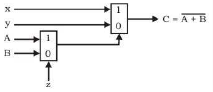Solution: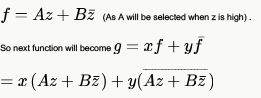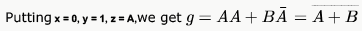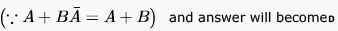QUESTION: 13

Suppose only one multiplexer and one inverter are allowed to be used to implement any Boolean function of  variables.
What is the minimum size of the multiplexer needed?

Solution:

2n - 1
We will map  variables  to select lines and  variable to input line

QUESTION: 14

The following circuit implements a two-input AND gate using two 2-1 multiplexers.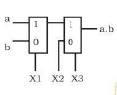What are the values of X1,X2,X3?

Solution:

F = (bX1' + aX1)X3 + X2X3'
Put X1 = b, X2 = 0, X3 = a to get F = ab.